Share

# HC Verma solutions for Class 12 Concepts of Physics Vol. 2 chapter 9 - Capacitors [Latest edition]

Textbook page

#### Chapters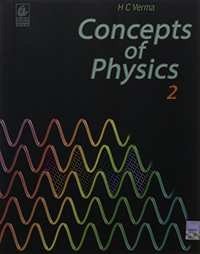## Chapter 9: Capacitors

#### HC Verma solutions for Class 12 Concepts of Physics Vol. 2 Chapter 9 Capacitors Exercise Short Answers [Page 163]

Short Answers | Q 1 | Page 163

Suppose a charge +Q1 is given to the positive plate and a charge −Q2 to the negative plate of a capacitor. What is the "charge on the capacitor"?

Short Answers | Q 2 | Page 163

As C = (1/V) Q , can you say that the capacitance C is proportional to the charge Q?

Short Answers | Q 3 | Page 163

A hollow metal sphere and a solid metal sphere of equal radii are given equal charges. Which of the two will have higher potential?

Short Answers | Q 4 | Page 163

The plates of a parallel-plate  capacitor are given equal positive charges. What will be the potential difference between the plates? What will be the charges on the facing surfaces and on the outer surfaces?

Short Answers | Q 5 | Page 163

A capacitor has capacitance C. Is this information sufficient to know what maximum charge the capacitor can contain? If yes, what is this charges? If no, what other information is needed?

Short Answers | Q 6 | Page 163

The dielectric constant decreases if the temperature is increased. Explain this in terms of polarization of the material.

Short Answers | Q 7 | Page 163

When a dielectric slab is gradually inserted between the plates of an isolated parallel-plate capacitor, the energy of the system decreases. What can you conclude about the force on the slab exerted by the electric field?

#### HC Verma solutions for Class 12 Concepts of Physics Vol. 2 Chapter 9 Capacitors Exercise MCQ [Page 164]

MCQ | Q 1 | Page 164

A capacitor of capacitance C is charged to a potential V. The flux of the electric field through a closed surface enclosing the capacitor is

• (CV)/∈_0

• (2CV)/∈_0

• (CV)/(2∈_0)

• Zero

MCQ | Q 2 | Page 164

Two capacitors each having capacitance C and breakdown voltage V are joined in series. The capacitance and the breakdown voltage of the combination will be

• 2 C and 2 V

• C/2 and V/2

• 2 C and V/2

• C/2 and 2 V.

MCQ | Q 3 | Page 164

If the capacitors in the previous question are joined in parallel, the capacitance and the breakdown voltage of the combination will be

• 2 C and 2 V

• C and 2 V

• 2 C and V

• C and V.

MCQ | Q 4 | Page 164

The equivalent capacitance of the combination shown in the figure is _________ .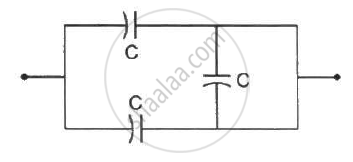• C

• 2 C

• C/2

• none of these.

MCQ | Q 5 | Page 164

A dielectric slab is inserted between the plates of an isolated capacitor. The force between the plates will

• increase

• decrease

• remain unchanged

• become zero

MCQ | Q 6 | Page 164

The energy density in the electric field created by a point charge falls off with the distance from the point charge as

• 1/r

• 1/r^2

• 1/r^3

• 1/r^4

MCQ | Q 7 | Page 164

A parallel-plate capacitor has plates of unequal area. The larger plate is connected to the positive terminal of the battery and the smaller plate to its negative terminal. Let Q, and Q be the charges appearing on the positive and negative plates respectively.

• Q, > Q

• Q, = Q

• Q, < Q

• The information is not sufficient is decide the relation between Q, and Q.

MCQ | Q 8 | Page 164

A thin metal plate P is inserted between the plates of a parallel-plate capacitor of capacitance C in such a way that its edges touch the two plates . The capacitance now becomes _________ .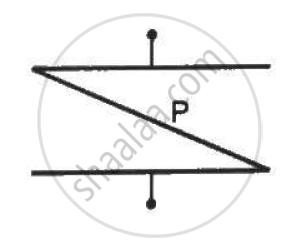• C/2

• 2 C

• 0

• ∞

MCQ | Q 9 | Page 164

The following figure shows two capacitors connected in series and joined to a battery. The graph shows the variation in potential as one moves from left to right on the branch containing the capacitors.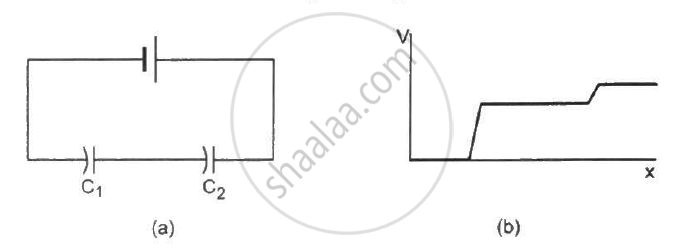• C1 > C2

• C1 = C2

• C1 < C2

• The information is not sufficient to decide the relation between C1 and C2.

MCQ | Q 10 | Page 164

Two metal plates having charges Q, −Q face each other at some separation and are dipped into an oil tank. If the oil is pumped out, the electric field between the plates will

• increase

• decrease

• remain the same

• become zero

MCQ | Q 11 | Page 164

Two metal spheres of capacitance C1 and C2 carry some charges. They are put in contact and then separated. The final charges Q1 and Q2 on them will satisfy

• Q_1/Q_2< C_1/C_2

• Q_1/Q_2 = C_1/C_2

• Q_1/Q_2 > C_1/C_2

• Q_1/Q_2 = C_2/C_1

MCQ | Q 12 | Page 164

Three capacitors of capacitances 6 µF each are available. The minimum and maximum capacitances, which may be obtained are

• 6 µF, 18 µF

• 3 µF, 12 µF

• 2 µF, 12 µF

• 2 µF, 18 µF

#### HC Verma solutions for Class 12 Concepts of Physics Vol. 2 Chapter 9 Capacitors Exercise MCQ [Pages 164 - 165]

MCQ | Q 1 | Page 164

The capacitance of a capacitor does not depend on

• the shape of the plates

• the size of the plates

• the charges on the plates

• the separation between the plates

MCQ | Q 2 | Page 164

A dielectric slab is inserted between the plates of an isolated charged capacitor. Which of the following quantities will remain the same?

• The electric field in the capacitor

• The charge on the capacitor

• The potential difference between the plates

• The stored energy in the capacitor

MCQ | Q 3 | Page 165

A dielectric slab is inserted between the plates of a capacitor. The charge on the capacitor is Q and the magnitude of the induced charge on each surface of the dielectric is Q'.

• Q' may be large than Q.

• Q' must be larger than Q.

• Q' must be equal to Q.

• Q' must be smaller than Q.

MCQ | Q 4 | Page 165

Each plate of a parallel plate capacitor has a charge q on it. The capacitor is now connected to a batter. Now,
(a) the facing surfaces of the capacitor have equal and opposite charges
(b) the two plates of the capacitor have equal and opposite charges
(c) the battery supplies equal and opposite charges to the two plates
(d) the outer surfaces of the plates have equal charges

MCQ | Q 5 | Page 165

The separation between the plates of a charged parallel-plate capacitor is increased. Which of the following quantities will change?
(a) Charge on the capacitor
(b) Potential difference across the capacitor
(c) Energy of the capacitor
(d) Energy density between the plates

MCQ | Q 6 | Page 165

A parallel-plate capacitor is connected to a battery. A metal sheet of negligible thickness is placed between the plates. The sheet remains parallel to the plates of the capacitor.

• The battery will supply more charge.

• The capacitance will increase.

• The potential difference between the plates will increase.

• Equal and opposite charges will appear on the two faces of the metal plate.

MCQ | Q 7 | Page 165

Following operations can be performed on a capacitor:
X − connect the capacitor to a battery of emf ε.
Y − disconnect the battery.
Z − reconnect the battery with polarity reversed.
W − insert a dielectric slab in the capacitor.
(a) In XYZ (perform X, then Y, then Z) the stored electric energy remains unchanged and no thermal energy is developed.
(b) The charge appearing on the capacitor is greater after the action XWY than after the action XYZ.
(c) The electric energy stored in the capacitor is greater after the action WXY than after the action XYW.
(d) The electric field in the capacitor after the action XW is the same as that after WX.

#### HC Verma solutions for Class 12 Concepts of Physics Vol. 2 Chapter 9 Capacitors [Pages 165 - 170]

Q 1 | Page 165

When 1⋅0 × 1012 electrons are transferred from one conductor to another, a potential difference of 10 V appears between the conductors. Calculate the capacitance of the two-conductor system.

Q 2 | Page 165

The plates of a parallel-plate capacitor are made of circular discs of radii 5⋅0 cm each. If the separation between the plates is 1⋅0 mm, what is the capacitance?

Q 3 | Page 165

Suppose, one wishes to construct a 1⋅0 farad capacitor using circular discs. If the separation between the discs be kept at 1⋅0 mm, what would be the radius of the discs?

Q 4 | Page 165

A parallel-plate capacitor having plate area 25 cm2 and separation 1⋅00 mm is connected to a battery of 6⋅0 V. Calculate the charge flown through the battery. How much work has been done by the battery during the process?

Q 5 | Page 165

A parallel-plate capacitor has plate area 25⋅0 cm2 and a separation of 2⋅00 mm between the plates. The capacitor is connected to a battery of 12⋅0 V. (a) Find the charge on the capacitor. (b) The plate separation is decreased to 1⋅00 mm. Find the extra charge given by the battery to the positive plate.

Q 6 | Page 165

Find the charges on the three capacitors connected to a battery as shown in figure.

Take C_1 = 2.0  uF , C_2 = 4.0  uF , C_3 = 6.0  uF and V = 12 volts.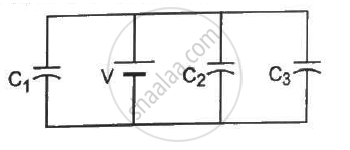Q 7 | Page 165

Three capacitors having capacitances 20 µF, 30 µF and 40 µF are connected in series with a 12 V battery. Find the charge on each of the capacitors. How much work has been done by the battery in charging the capacitors?

Q 8 | Page 165

Find the charge appearing on each of the three capacitors shown in figure .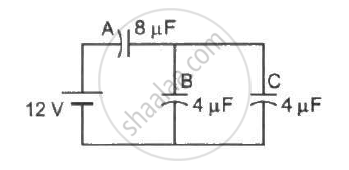Q 9 | Page 165

Take C_1 = 4.0  "uF" and C_2 = 6.0  "uF" in figure . Calculate the equivalent capacitance of the combination between the points indicated.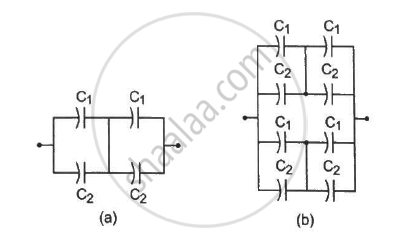Q 10 | Page 166

Find the charge supplied by the battery in the arrangement shown in figure.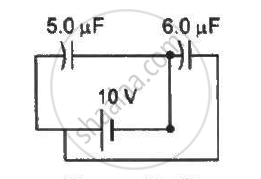Q 11 | Page 166

The outer cylinders of two cylindrical capacitors of capacitance 2⋅2 µF each, are kept in contact and the inner cylinders are connected through a wire. A battery of emf 10 V is connected as shown in figure . Find the total charge supplied by the battery to the inner cylinders.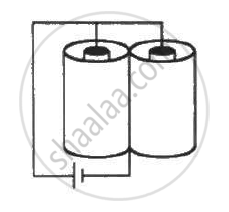Q 12 | Page 166

Two conducting spheres of radii R1 and R2 are kept widely separated from each other. What are their individual capacitances? If the spheres are connected by a metal wire, what will be the capacitance of the combination? Think in terms of series−parallel connections.

Q 13 | Page 166

Each of the capacitors shown in figure has a capacitance of 2 µF. find the equivalent capacitance of the assembly between the points A and B. Suppose, a battery of emf 60 volts is connected between A and B. Find the potential difference appearing on the individual capacitors.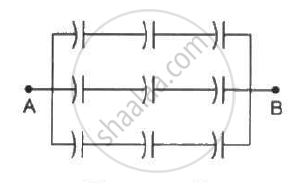Q 14 | Page 166

It is required to construct a 10 µF capacitor which can be connected across a 200 V battery. Capacitors of capacitance 10 µF are available but they can withstand only 50 V. Design a combination which can yield the desired result.

Q 15 | Page 166

Take the potential of the point B in figure to be zero. (a) Find the potentials at the points C and D. (b) If a capacitor is connected between C and D, what charge will appear on this capacitor?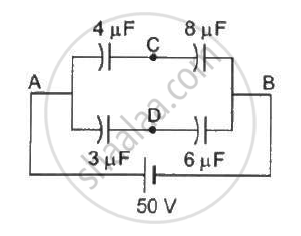Q 16 | Page 166

Find the equivalent capacitance of the system shown in figure between the points a and b.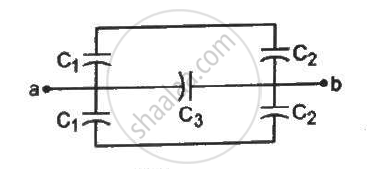Q 17 | Page 166

A capacitor is made of a flat plate of area A and a second plate having a stair-like structure as shown in figure . The width of each stair is a and the height is b. Find the capacitance of the assembly.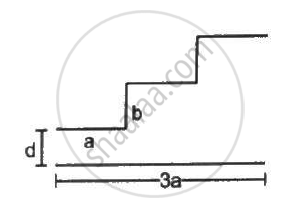Q 18 | Page 166

A cylindrical capacitor is constructed using two coaxial cylinders of the same length 10 cm and of radii 2 mm and 4 mm. (a) Calculate the capacitance. (b) Another capacitor of the same length is constructed with cylinders of radii 4 mm and 8 mm. Calculate the capacitance.

Q 19 | Page 166

A 100 pF capacitor is charged to a potential difference of 24 V. It is connected to an uncharged capacitor of capacitance 20 pF. What will be the new potential difference across the 100 pF capacitor?

Q 20 | Page 166

Each capacitor shown in figure has a capacitance of 5⋅0 µF. The emf of the battery is 50 V. How much charge will flow through AB if the switch S is closed?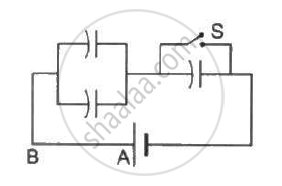Q 21 | Page 166

The particle P shown in figure has a mass of 10 mg and a charge of −0⋅01 µC. Each plate has a surface area 100 cm2 on one side. What potential difference V should be applied to the combination to hold the particle P in equilibrium?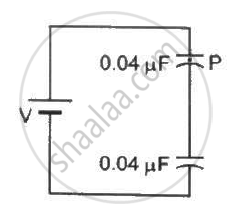Q 22 | Page 166

Both the capacitors shown in figure are made of square plates of edge a. The separations between the plates of the capacitors are d1 and d2 as shown in the figure. A potential difference V is applied between the points a and b. An electron is projected between the plates of the upper capacitor along the central line. With what minimum speed should the electron be projected so that it does not collide with any plate? Consider only the electric forces.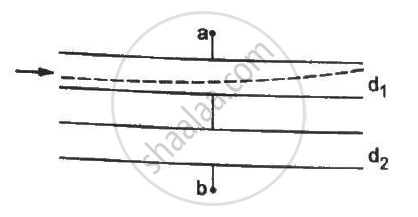Q 23 | Page 167

The plates of a capacitor are 2⋅00 cm apart. An electron-proton pair is released somewhere in the gap between the plates and it is found that the proton reaches the negative plate at the same time as the electron reaches the positive plate. At what distance from the negative plate was the pair released?

Q 24 | Page 167

Convince yourself that parts (a), (b) and (c) figure are identical. Find the capacitance between the points A and B of the assembly.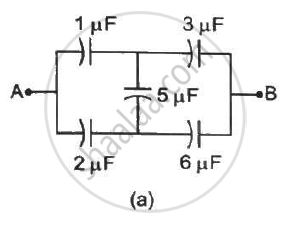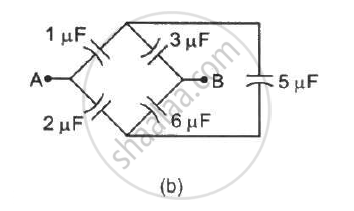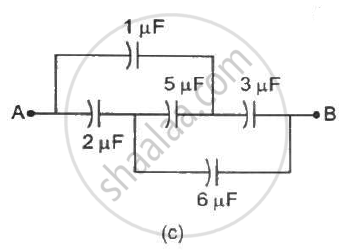Q 25 | Page 167

Find the potential difference V_a - V_b between the points a and b shown in each part of the figure.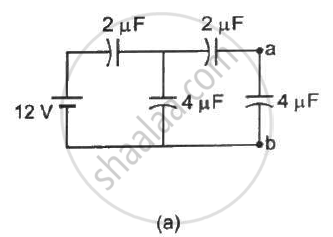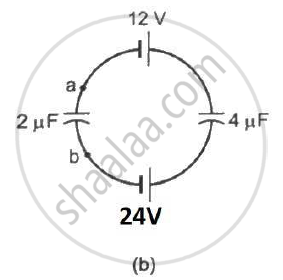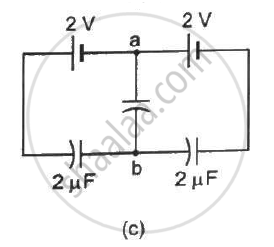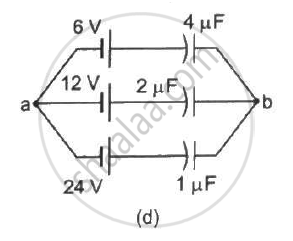Q 26 | Page 167

Find the equivalent capacitances of the combinations shown in figure between the indicated points.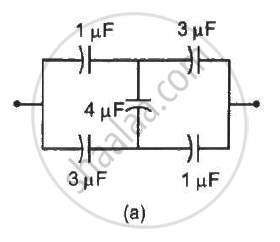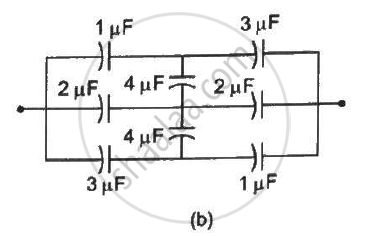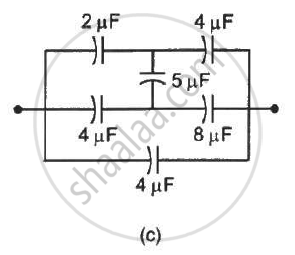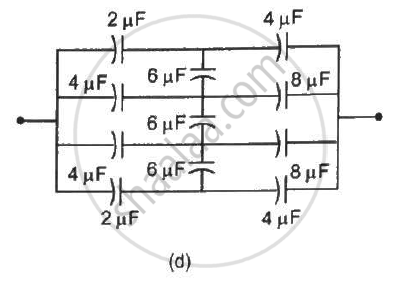Q 27 | Page 167

Find the capacitance of the combination shown in figure between A and B.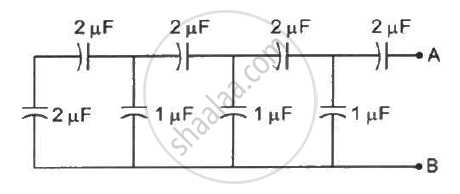Q 28 | Page 167

Find the equivalent capacitance of the infinite ladder shown in figure between the points A and B.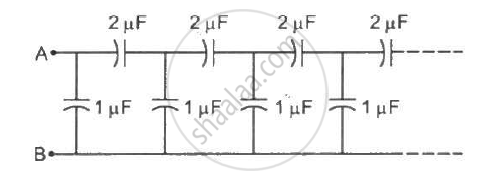Q 29 | Page 167

A finite ladder is constructed by connecting several sections of 2 µF, 4 µF capacitor combinations as shown in the figure. It is terminated by a capacitor of capacitance C. What value should be chosen for C, such that the equivalent capacitance of the ladder between the points A and B becomes independent of the number of sections in between?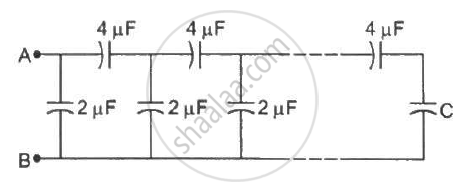Q 30 | Page 167

A charge of +2.0 xx 10^-8 C  is placed on the positive plate and a charge of -1.0 xx 10^-8 C on the negative plate of a parallel-plate capacitor of capacitance 1.2 xx 10^-3  "uF" . Calculate the potential difference developed between the plates.

Q 31 | Page 168

A charge of 20 µC is placed on the positive plate of an isolated parallel-plate capacitor of capacitance 10 µF. Calculate the potential difference developed between the plates.

Q 32 | Page 168

A charge of 1 µC is given to one plate of a parallel-plate capacitor of capacitance 0⋅1 µF and a charge of 2 µC is given to the other plate. Find the potential difference developed between the plates.

Q 33 | Page 168

Each of the plates shown in figure has surface area (96/∈_0) xx 10^-12 Fm on one side and the separation between the consecutive plates is 4⋅0 mm. The emf of the battery connected is 10 volts. Find the magnitude of the charge supplied by the battery to each of the plates connected to it.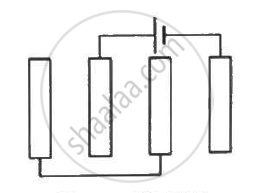Q 34 | Page 168

The capacitance between the adjacent plates shown in figure is 50 nF. A charge of 1⋅0 µC is placed on the middle plate. (a) What will be the charge on the outer surface of the upper plate? (b) Find the potential difference developed between the upper and the middle plates.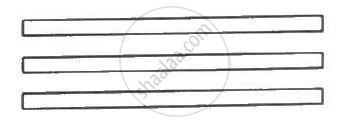Q 35 | Page 168

The capacitance between the adjacent plates shown in the figure is 50 nF. A charge of 1.0µC is placed on the middle plate. If 1.0 µC is placed on the upper plate instead of the middle, what will be the potential difference between (a) the upper and the middle plates and (b) the middle and the lower plates?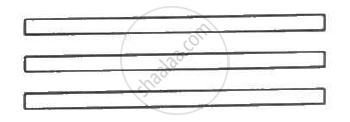Q 36 | Page 168

Two capacitors of capacitance 20⋅0 pF and 50⋅0 pF are connected in series with a 6⋅00 V battery. Find (a) the potential difference across each capacitor and (b) the energy stored in each capacitor.

Q 37 | Page 168

Two capacitors of capacitances 4⋅0 µF and 6⋅0 µF are connected in series with a battery of 20 V. Find the energy supplied by the battery.

Q 38 | Page 168

Each capacitor in figure has a capacitance of 10 µF. The emf of the battery is 100 V. Find the energy stored in each of the four capacitors.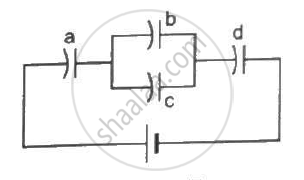Q 39 | Page 168

A capacitor with stored energy 4⋅0 J is connected with an identical capacitor with no electric field in between. Find the total energy stored in the two capacitors.

Q 40 | Page 168

A capacitor of capacitance 2⋅0 µF is charged to a potential difference of 12 V. It is then connected to an uncharged capacitor of capacitance 4⋅0 µF as shown in figure . Find (a) the charge on each of the two capacitors after the connection, (b) the electrostatic energy stored in each of the two capacitors and (c) the heat produced during the charge transfer from one capacitor to the other.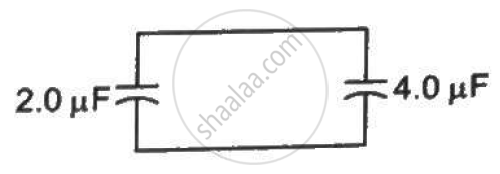Q 41 | Page 168

A point charge Q is placed at the origin. Find the electrostatic energy stored outside the sphere of radius R centred at the origin.

Q 42 | Page 168

A metal sphere of radius R is charged to a potential V. (a) Find the electrostatic energy stored in the electric field within a concentric sphere of radius 2 R. (b) Show that the electrostatic field energy stored outside the sphere of radius 2 R equals that stored within it.

Q 43 | Page 168

A large conducting plane has a surface charge density 1.0 xx 10^-4  "Cm"^-2 . Find the electrostatic energy stored in a cubical volume of edge 1⋅0 cm in front of the plane.

Q 44 | Page 168

A parallel-plate capacitor having plate area 20 cm2 and separation between the plates 1⋅00 mm is connected to a battery of 12⋅0 V. The plates are pulled apart to increase the separation to 2⋅0 mm. (a) Calculate the charge flown through the circuit during the process. (b) How much energy is absorbed by the battery during the process? (c) Calculate the stored energy in the electric field before and after the process. (d) Using the expression for the force between the plates, find the work done by the person pulling the plates apart. (e) Show and justify that no heat is produced during this transfer of charge as the separation is increased.

Q 45 | Page 168

A capacitor having a capacitance of 100 µF is charged to a potential difference of 24 V. The charging battery is disconnected and the capacitor is connected to another battery of emf 12 V with the positive plate of the capacitor joined with the positive terminal of the battery. (a) Find the charges on the capacitor before and after the reconnection. (b) Find the charge flown through the 12 V battery. (c) Is work done by the battery or is it done on the battery? Find its magnitude. (d) Find the decrease in electrostatic field energy. (e) Find the heat developed during the flow of charge after reconnection.

Q 46 | Page 168

Consider the situation shown in the figure. The switch S is open for a long time and then closed. (a) Find the charge flown through the battery when the switch S is closed. (b) Find the work done by the battery.(c) Find the change in energy stored in the capacitors.(d) Find the heat developed in the system.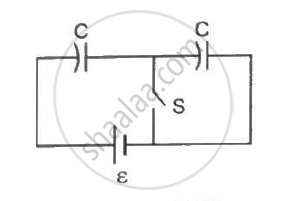Q 47 | Page 169

A capacitor of capacitance 5⋅00 µF is charged to 24⋅0 V and another capacitor of capacitance 6⋅0 µF is charged to 12⋅0 V. (a) Find the energy stored in each capacitor. (b) The positive plate of the first capacitor is now connected to the negative plate of the second and vice versa. Find the new charges on the capacitors. (c) Find the loss of electrostatic energy during the process. (d) Where does this energy go?

Q 48 | Page 168

A 5⋅0 µF capacitor is charged to 12 V. The positive plate of this capacitor is now connected to the negative terminal of a 12 V battery and vice versa. Calculate the heat developed in the connecting wires.

Q 49 | Page 169

The two square faces of a rectangular dielectric slab (dielectric constant 4⋅0) of dimensions 20 cm × 20 cm × 1⋅0 mm are metal-coated. Find the capacitance between the coated surfaces.

Q 50 | Page 169

If the above capacitor is connected across a 6⋅0 V battery, find (a) the charge supplied by the battery, (b) the induced charge on the dielectric and (c) the net charge appearing on one of the coated surfaces.

Q 51 | Page 169

The separation between the plates of a parallel-plate capacitor is 0⋅500 cm and its plate area is 100 cm2. A 0⋅400 cm thick metal plate is inserted into the gap with its faces parallel to the plates. Show that the capacitance of the assembly is independent of the position of the metal plate within the gap and find its value.

Q 52 | Page 169

A capacitor stores 50 µC charge when connected across a battery. When the gap between the plates is filled with a dielectric, a charge of 100 µC flows through the battery. Find the dielectric constant of the material inserted.

Q 53 | Page 169

A parallel-plate capacitor of capacitance 5 µF is connected to a battery of emf 6 V. The separation between the plates is 2 mm. (a) Find the charge on the positive plate. (b) Find the electric field between the plates. (c) A dielectric slab of thickness 1 mm and dielectric constant 5 is inserted into the gap to occupy the lower half of it. Find the capacitance of the new combination. (d) How much charge has flown through the battery after the slab is inserted?

Q 54 | Page 169

A parallel-plate capacitor has plate area 100 cm2 and plate separation 1⋅0 cm. A glass plate (dielectric constant 6⋅0) of thickness 6⋅0 mm and an ebonite plate (dielectric constant 4⋅0) are inserted one over the other to fill the space between the plates of the capacitor. Find the new capacitance.

Q 55 | Page 169

A parallel-plate capacitor having plate area 400 cm2 and separation between the plates 1⋅0 mm is connected to a power supply of 100 V. A dielectric slab of thickness 0⋅5 mm and dielectric constant 5⋅0 is inserted into the gap. (a) Find the increase in electrostatic energy. (b) If the power supply is now disconnected and the dielectric slab is taken out, find the further increase in energy. (c) Why does the energy increase in inserting the slab as well as in taking it out?

Q 56 | Page 169

Find the capacitances of the capacitors shown in figure . The plate area is Aand the separation between the plates is d. Different dielectric slabs in a particular part of the figure are of the same thickness and the entire gap between the plates is filled with the dielectric slabs.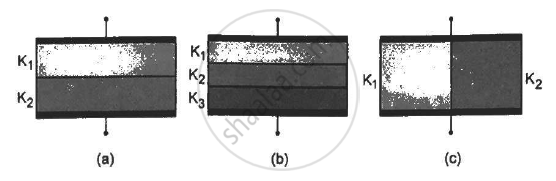Q 57 | Page 169

A capacitor is formed by two square metal-plates of edge a, separated by a distance d. Dielectrics of dielectric constant K1 and K2 are filled in the gap as shown in figure . Find the capacitance.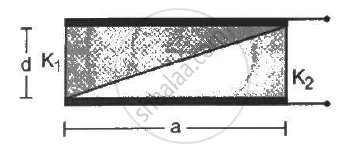Q 58 | Page 169

Figure shows two identical parallel plate capacitors connected to a battery through a switch S. Initially, the switch is closed so that the capacitors are completely charged. The switch is now opened and the free space between the plates of the capacitors is filled with a dielectric of dielectric constant 3. Find the ratio of the initial total energy stored in the capacitors to the final total energy stored.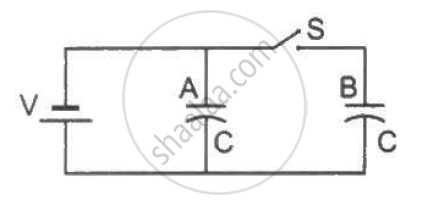Q 59 | Page 169

A parallel-plate capacitor of plate area A and plate separation d is charged to a potential difference V and then the battery is disconnected. A slab of dielectric constant K is then inserted between the plates of the capacitor so as to fill the space between the plates. Find the work done on the system in the process of inserting the slab.

Q 60 | Page 169

A capacitor having a capacitance of 100 µF is charged to a potential difference of 50 V. (a) What is the magnitude of the charge on each plate? (b) The charging battery is disconnected and a dielectric of dielectric constant 2⋅5 is inserted. Calculate the new potential difference between the plates. (c) What charge would have produced this potential difference in absence of the dielectric slab. (d) Find the charge induced at a surface of the dielectric slab.

Q 61 | Page 169

A sphercial capacitor is made of two conducting spherical shells of radii a and b. The space between the shells is filled with a dielectric of dielectric constant K up to a radius c as shown in figure . Calculate the capacitance.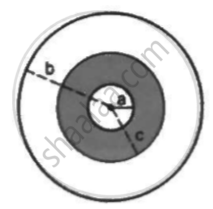Q 62 | Page 170

Consider an assembly of three conducting concentric spherical shell of radii a, b and c as shown in figure Find the capacitance of the assembly between the points Aand B.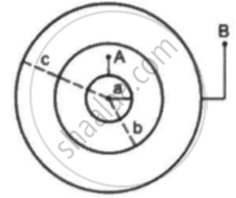Q 63 | Page 170

Suppose the space between the two inner shells is filled with a dielectric of dielectric constant K. Find the capacitance of the system between A and B.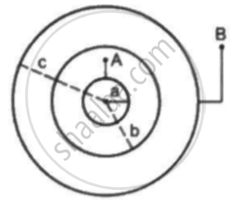Q 64 | Page 170

An air-filled parallel-plate capacitor is to be constructed which can store 12 µC of charge when operated at 1200 V. What can be the minimum plate area of the capacitor? The dielectric strength of air is 3 xx 10^6  "Vm"^-1

Q 65 | Page 170

A parallel-plate capacitor with the plate area 100 cm2 and the separation between the plates 1⋅0 cm is connected across a battery of emf 24 volts. Find the force of attraction between the plates.

Q 66 | Page 170

Consider the situation shown in figure (31-E29). The width of each plate is b. The capacitor plates are rigidly clamped in the laboratory and connected to a battery of emf ε. All surfaces are frictionless. Calculate the value of M for which the dielectric slab will stay in equilibrium.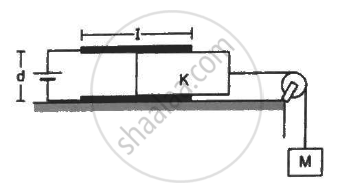Q 67 | Page 170

Figure shows two parallel plate capacitors with fixed plates and connected to two batteries. The separation between the plates is the same for the two capacitors. The plates are rectangular in shape with width b and lengths l1 and l2. The left half of the dielectric slab has a dielectric constant K1 and the right half K2. Neglecting any friction, find the ration of the emf of the left battery to that of the right battery for which the dielectric slab may remain in equilibrium.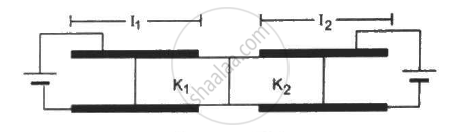Q 68 | Page 170

Consider the situation shown in figure. The plates of the capacitor have plate area A and are clamped in the laboratory. The dielectric slab is released from rest with a length a inside the capacitor. Neglecting any effect of friction or gravity, show that the slab will execute periodic motion and find its time period.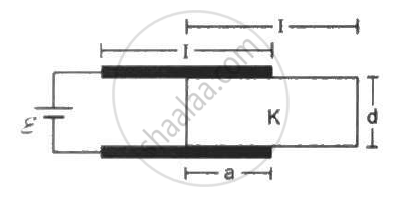## Chapter 9: Capacitors## HC Verma solutions for Class 12 Concepts of Physics Vol. 2 chapter 9 - Capacitors

HC Verma solutions for Class 12 Concepts of Physics Vol. 2 chapter 9 (Capacitors) include all questions with solution and detail explanation. This will clear students doubts about any question and improve application skills while preparing for board exams. The detailed, step-by-step solutions will help you understand the concepts better and clear your confusions, if any. Shaalaa.com has the CBSE Class 12 Concepts of Physics Vol. 2 solutions in a manner that help students grasp basic concepts better and faster.

Further, we at Shaalaa.com provide such solutions so that students can prepare for written exams. HC Verma textbook solutions can be a core help for self-study and acts as a perfect self-help guidance for students.

Concepts covered in Class 12 Concepts of Physics Vol. 2 chapter 9 Capacitors are Combination of Capacitors, Capacitors and Capacitance, Dielectrics and Polarisation, Free Charges and Bound Charges Inside a Conductor, Conductors and Insulators Related to Electric Field, Electrical Potential Energy of a System of Two Point Charges and of Electric Dipole in an Electrostatic Field, Equipotential Surfaces, Potential Due to a System of Charges, Electric Potential Difference, Potential Due to a Point Charge, Electric Potential, Van De Graaff Generator, Effect of Dielectric on Capacity, The Parallel Plate Capacitor, Electrostatics of Conductors, Potential Energy of a Dipole in an External Field, Potential Energy of a System of Two Charges in an External Field, Potential Energy of a Single Charge, Potential Energy of a System of Charges, Potential Due to an Electric Dipole, Relation Between Electric Field and Electrostatic Potential, Energy Stored in a Capacitor, Capacitance of a Parallel Plate Capacitor with and Without Dielectric Medium Between the Plates.

Using HC Verma Class 12 solutions Capacitors exercise by students are an easy way to prepare for the exams, as they involve solutions arranged chapter-wise also page wise. The questions involved in HC Verma Solutions are important questions that can be asked in the final exam. Maximum students of CBSE Class 12 prefer HC Verma Textbook Solutions to score more in exam.

Get the free view of chapter 9 Capacitors Class 12 extra questions for Class 12 Concepts of Physics Vol. 2 and can use Shaalaa.com to keep it handy for your exam preparation

S